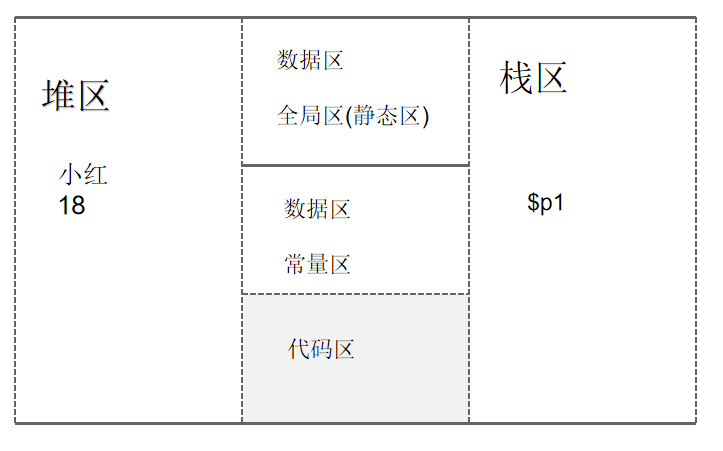PHP入门之类型与运算符
PHP入门之流程控制
PHP入门之函数
PHP入门之数组
PHP基础之排序
PHP基础之查找

# 类与对象基本概念

<?php
//创建一个对象
class cat {
public $name; public$age;
public $color; } //创建一个猫$cat1= new cat;
$cat1->name="小刘";$cat1->age=18;
$cat1->color="yellow"; //再创建一个猫$cat2= new cat;
$cat2->name="小陈";$cat2->age=16;
$cat2->color="pink"; //输出两个猫的信息 if ($cat1->name="小刘"){
echo $cat1->name."||".$cat1->age."||".$cat1->color.'<br/>'; }if ($cat2->name="小陈"){
echo $cat2->name."||".$cat2->age."||".$cat2->color; } ?>  总结几句话： • ①类是抽象的，代表一类事物。 • ②对象是具体，是类的一个具体实例。 • ③类是对象的模板，对象是类的一个个具体实例。 类的基本格式 class 类名{ 成员属性(变量); } 成员属性是从某个事物提取出来的，它可以是 基本数据类型，也可以是复合数据类型(数组，对象) 如何创建对象？$对象名=new 类名();
$对象名=new 类名; //两种方式都可以 对象如何访问(使用)对象的属性？$对象名->属性名;

# 对象在内存中存在形式

<?php
class Person {
public $name; public$age;
}
$p1= new Person();$p1->name="小红";
$p1->age=18;$p2=$p1; echo$p1->name.'<br/>';
echo $p2->age.'<br/>'; ?>  现在画一下内存图：name="小红";age=18;变量$p1->name和age时就会由栈区指向堆区。

<?php
class Person {
public $name; public$age;
}
$p1= new Person();$p1->name="小红";
$p1->age=18; #我们发现输出结果为大红，所以，函数接收对象时候，接收得是地址。 function test ($p){
$p->name="大红"; } test($p1);
echo $p1->name.'<br/>'; ?>  如果给函数传递的是基本数据类型(整行，浮点型，布尔型)，传递的是什么？ 默认情况下传递的是值。如果希望传地址，那就加上&符。 如果给一个函数传递的是一个数组，则默认情况下是传值。 举个例子： <?php$arr=array($a1,$a2);
$a1=array(3,5,8);$a2=array(5,7,9);
var_dump($arr); ?>  可以输出结果吗？答案是无法输出结果。会报变量没有定义的错误。因为是传值，所以第一行的$a1和第二行的$a1是两码事。 如果换一下顺序，就可以了。 <?php$a1=array(3,5,8);
$a2=array(5,7,9);$arr=array($a1,$a2);
var_dump($arr); ?>  这样就可以输出了，数组有值了。 # 构造函数 什么是构造函数（方法）？ 想要知道什么是构造函数，我们先看一个需求，之前我们创建一个对象的时候，是创建好之后，再给对象的属性进行赋值，如果我们再创建对象的时候就直接给属性赋值，这样该如何做呢？下面我们就要引入构造函数了。 上面的问题，我们只需要定义一个构造函数就可以了。构造函数是类的一种特殊的函数，它的主要作用是完成对新对象的初始化。 构造函数特点： ①没有返回值。 ②在创建一个类的新对象时，系统会自动的调用该类的构造函数完成对新对象的初始化。 用一个小案例说明： <?php class Person{ public$name;
public $age; function __construct($iname,$iage) {$name=$iname;$age=$iage; echo "我是构造函数"; echo '<br/>'; } }$p1=new Person("小可爱",18);
echo $p1->name; echo '<br/>'; echo$p1->age;
?>


        $name=$iname;
$age=$iage;


    $this->name=$iname;
$this->age=$iage;


function __construct(){}

public $age; //构造函数 function __construct($name,$age) {$this->name=$name;$this->age=$age; } //析构函数 function __destruct() { // TODO: Implement __destruct() method. echo$this->name.'销毁资源'.'<br/>';
}
}
$p1= new Person("小王",18);$p2=new Person("小张",20);
?>


• 一、程序运行完退出的时候。
• 二、当对象没有变量指向它的时候，它会变成垃圾对象，会立刻调用析构函数回收。(和Java不一样)。
还有两点需要注意：
• 一、析构函数没有返回值。
• 二、一个类最多只能有一个析构函数。

# 静态变量与静态方法

<?php
global $child_sums;$child_sums=0;
class Child
{
public $name; function __construct($name)
{
$this->name =$name;
}

function JoinChild()
{
//申明使用全局变量
global $child_sums;$child_sums+=1;
echo $this->name . "加入游戏"; } } //创建三个小孩$child1=new Child("拉拉");
$child1->JoinChild();$child2=new Child("哈哈");
$child2->JoinChild();$child3=new Child("哒哒");
$child3->JoinChild(); echo "<br/>"."有".$child_sums."个小朋友";
?>


<?php
class Child{
public $name; public static$sums=0;
//构造函数
function __construct($name) {$this->name=$name; } function JoinChild(){ self::$sums+=1;
echo $this->name.'加入游戏'; } } //创建三个小孩$child1=new Child("拉拉");
$child1->JoinChild();$child2=new Child("哈哈");
$child2->JoinChild();$child3=new Child("哒哒");
$child3->JoinChild(); //看看多少人 echo '<br/>'."一共".Child::$sums."个小孩";
?>


## 静态方法

<?php
class Child{
public $name; public static$sums=0;
//构造函数
function __construct($name) {$this->name=$name; } static function JoinChild(){ //self::$sums+=1;
Child::$sums+=1; } function haizi(){ echo$this->name;
}
}
//创建三个小孩
$child1=new Child("拉拉");$child1->haizi();
$child1->JoinChild();$child2=new Child("哈哈");
$child2->haizi();$child1->JoinChild();
$child3=new Child("哒哒");$child3->haizi();
$child1->JoinChild(); //看看多少人 echo '<br/>'."一共".Child::$sums."个小孩";
?>


   static function JoinChild(){
//self::$sums+=1; Child::$sums+=1;
}


# 面向对象三大特性之封装

public(公开的)、protected(受保护的)、private(私有的)

<?php
class Person{
public $name; protected$age;
private $wage; public function __construct($name,$age,$wage)
{
$this->name=$name;
$this->age=$age;
$this->wage=$wage;

}
}
$p1=new Person("小利",18,1000); echo$p1->name;
echo $p1->age; #报错 echo$p1->wage; #报错
?>


    public function PersonAge($age){ echo$this->age=$age; } public function PersonWage($wage){
echo $this->wage=$wage;
}


$p1->PersonAge(20);$p1->PersonWage(3000);


__set()对protected或是private属性，进行赋值操作。
__get()获取protected或是private属性的值。

# 面向对象三大特性之继承

<?php
//父类
class Student{
public $name; public$age;
public $studentID; public function ShowInfo($name,$age){ echo$this->name=$name."||".$this->age=$age; } } //子类 class universityStudent extends Student{ public function study(){ echo "大学生在学习"; } }$u1=new universityStudent();
$u1->ShowInfo("小练习",18);$u1->study();
?>


<?php
class Student{
public $name; public$age;
public $studentID; function __construct() { echo "我是父类的构造函数"."<br/>"; } public function ShowInfo($name,$age){ echo$this->name=$name."||".$this->age=$age; } } class universityStudent extends Student{ public function __construct() { echo "我是子类的构造函数"."<br/>"; } public function study(){ echo "大学生在学习"; } }$u1=new universityStudent();
$u1->ShowInfo("小练习",18);$u1->study();
?>


我是子类的构造函数



    public function __construct()
{
Student::__construct();
echo "我是子类的构造函数"."<br/>";
}


我是父类的构造函数



# 面向对象三大特性之多态

## 函数重载

“重载”是类的多态的一种实现，是指的是一个标识符被用作多个函数名，并且能够通过参数个数或者参数类型将这些同名的函数区分开，调用不发生混淆。
PHP虽然支持重载，但重载在具体实现上，和其他语言有较大的差别。举个例子：

class A{
public $name; public$age;
public function test(){
echo "hello,123";
}
public function test($a){ #如果我们这么写，PHP会报错！！！！其他的语言可以，Java这么写的话没问题。 echo "hello,456"; } }$a=new A();
$a->test();$a->test($a);  上面的是错误的写法。PHP有自己的方法，这里PHP引进了魔术方法。魔术方法：__call() 这个方法比较神奇。下面看代码： class A{ public$name;
public $age; public function test1($a){
echo "hello,123";
}
public function test2($a){ echo "hello,456"; } public function __call($name, $arguments) { var_dump($arguments);
if($name=="test"){ if(count($arguments)==1){
$this->test1($arguments);
}elseif (count($arguments)==2){$this->test2($arguments); } } // TODO: Implement __call() method. } }$a=new A();
$a->test(1);$a->test(2,6);
/*执行结果为：
array(1) { => int(1) } hello,123array(2) { => int(2) => int(6) } hello,456



echo "<br/>".__LINE__;
echo "<br/>".__DIR__;
echo "<br/>".__FILE__;
echo "<br/>".__CLASS__;
echo "<br/>".__TRAIT__;
echo "<br/>".__FUNCTION__;
echo "<br/>".__METHOD__;
echo "<br/>".__NAMESPACE__;

150
D:\phpstudy_pro\WWW\PHP
D:\phpstudy_pro\WWW\PHP\object02.php
A
test1
A::test1
array(2) { => int(2) => int(6) } hello,456


# 抽象类

abstract class 类名{
abstract 修饰符 function 函数名(参数列表)；//这里要注意，没有方法体。
}

• 抽象类不能被实例化。
• 抽象类不一定要包含abstract方法。也就是说，抽象类可以没有abstract方法。
• 一旦类包含了abstract方法，则这个类必须声明为abstract。
• 抽象方法不能有函数体。
• 如果一个类继承了某个抽象类，则它必须实现该抽象类的所有抽象方法(除非自己也声明为抽象类)。

# 接口

<?php
interface iTest{
public function start();
public function stop();
}
class camera implements iTest{
public function start(){
echo "相机开始工作";
}
public function stop(){
echo "相机停止工作";
}
}
class phone implements iTest{
public function start(){
echo "手机开始工作";
}
public function stop(){
echo "手机停止工作";
}
}
$c1=new camera();$c1->start();
$c1->stop(); echo "<br/>";$p1=new phone();
$p1->start();$p1->stop();
?>


public class A implements 接口1，接口2{
}

interface 接口名 extends 接口1，接口2{
}

final不能用来修饰成员属性。

## const概念

const 常量名=值;

echo '<br/>继续执行';
?>


Warning: fopen(123.txt): failed to open stream: No such file or directory in D:\phpstudy_pro\WWW\PHP\error.php on line 2

<?php
/*$fp=fopen("123.txt","r"); echo '<br/>继续执行';*/ if (!file_exists("abc.txt")){ echo "文件不存在!!"; exit(); }else{$fp=fopen("abc.txt","r");
echo "文件打开成功";
fclose(\$fp);  //这个必须有！！！

}
?>


<?php
if (!file_exists("abc.txt")){
die("文件不存在！");
}else{
//文件处理。。。。
}
?>


file_exists("abc.txt") or die("文件不存在！！！！");
#文件存在向下执行，不存在得话执行die()


# 小结

posted @ 2020-09-19 18:46  雪痕*  阅读(127)  评论(0编辑  收藏